# Chapter 17 Constructions RD Sharma Solutions Exercise 17.2 Class 9 Maths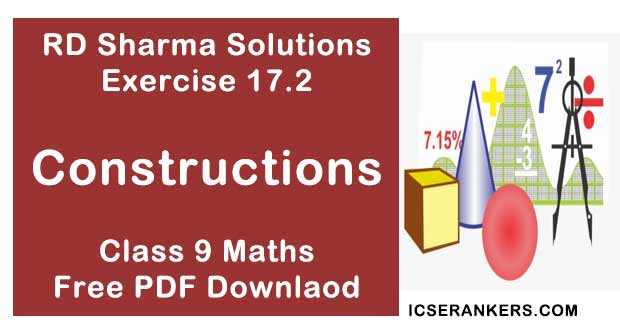Chapter Name RD Sharma Chapter 17 Constructions Exercise 17.2 Book Name RD Sharma Mathematics for Class 10 Other Exercises Exercise 17.1Exercise 17.3 Related Study NCERT Solutions for Class 10 Maths

### Exercise 17.2 Solutions

1. Draw an angle and label it as ∠BAC. Construct another angle, equal to ∠BAC.

Solution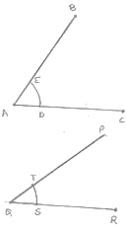Steps of construction :
1. Draw an angle ABO and a Line segment QR
2. With center A and any radius, draw an arc which intersects ∠BAC at E and O
3. With center Q and same radius draw arc which intersect QR at S.
4. With center S and radius equal to DE, draw an arc which intersect previous arc at T
5. Draw a line segment joining Q and T
∴ ∠PQR = ∠BAC

2. Draw an obtuse angle, Bisect it. Measure each of the angles so obtained.
Solution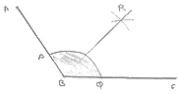Steps of construction :
1. Draw angle ABC of 120°
2. With center B and any radius, draw an arc which intersects AB at P and BC at Q
3. With center P and Q and radius more than 1/2 PQ, draw two arcs, with intersect each other at R.
4. Join BR
∴ ∠ABR = ∠RBC = 60°

3. Using your protractor, draw an angle of measure 108° . With this angle as given, draw an angle of 54° .
Solution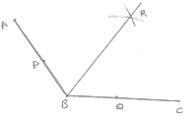Steps of construction :
1. Draw an angle ABC of 108°
2. With center B and any radius, draw an arc which intersects AB at P and BC at Q
3. With center P and Q and radius more than 1/2 PQ, draw two arcs, which intersect each other at R.
4. Join BR
∴ ∠RBC = 54°

4. Using protractor, draw a right angle. Bisect it to get an angle of measure 45° .
Solution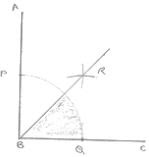Steps of construction :
1. Draw an angle ABC of 90°
2. With center B and any radius, draw an arc which intersects AB at P and BC at Q
3. With center P and Q and radius more than  1/2 PQ, draw two arcs, which intersect each other at R.
4. Join RB
∴ ∠RBC = 45°

5. Draw a linear pair of angles. Bisect each of the two angles. Verify that the two bisecting rays are perpendicular to each other.
Solution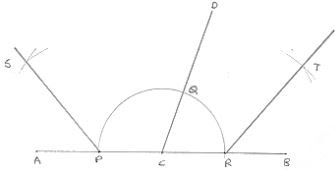Steps of construction :
1. Draw two angle DCA and DCB forming Linear pair.
2. With center C and any radius, draw an arc which intersects AC at P, CD at Q and CB at R.
3. With center P and Q and any radius draw two arcs which intersect each other at S
4. Join SC
5. With center Q and R any radius draw two arcs, which intersect each other at T.
6. Join TC
∠SCT = 90° [By using protractor]

6. Draw a pair of vertically opposite angles. Bisect each of the two angles. Verify that the bisecting rays are in the same line.
Solution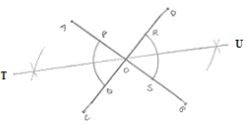Steps of construction :
1. Draw a pair of vertically opposite angle AOC and DOB
2. With center O and any radius drawn two arcs which intersect OA at P, Q. OB at S and OD at R.
3. With center P and Q and radius more than 1/2 PQ, draw two arcs which intersect each other at 7.
4. Join TO
5. With center R and S radius more than 1/2 RS, draw two arcs which intersect each other at U.
6. Join OU.
∴ TOU is a straight line

7. Using ruler and compasses only, draw a right angle.
Solution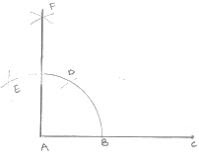Steps of construction :
1. Draw a line segment AB
2. With center A and any radius draw arc which intersect AB at C.
3. With center C and same radius draw an arc which intersects AB at C.
4. With center D and same radius draw arc which intersect arc in (2) at E.
5. With centers E and C and any radius, draw two arcs which intersect each other at F.
6. Join FA
∠FAB = 90°

8. Using ruler and compasses only, draw an angle of measure 135° .
Solution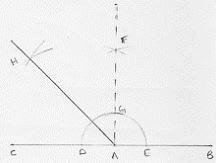Steps of construction :
1. Draw a line segment AB and produce BA to point C.
2. With center A and any radius draw arc which intersect AC at D and AB at E.
3. With center D and E and radius more than 1/2 DE, draw two arcs which intersect each other at F.
4. Join FA which intersect the arc in (2) at G.
5. With centers G and D and radius more than 1/2 GD, draw two arcs which intersect each other a H.
6. Join HA
∴ ∠HAB = 135°

9. Using a protractor, draw an angle of measure 72° . With this angle as given, draw angles of measure 36° and 54°.
Solution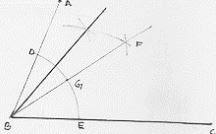Steps of construction :
1. Draw an angle ABC of 72° with the help of protractor.
2. With center B and any radius, draw an arc which intersect AB at D and BC at E.
3. With center D and E and radius more than 1/2 DE, draw two arcs which intersect each other at F.
4. Join FB which intersect the arc in (2) at G.
5. With centers D and G and radius more than 1/2 DE, draw two arcs which intersect each other at F.
6. With centers D and G and radius more thandraw two arcs which intersect each other at H.
7. Join HB
∴ ∠HBC = 54°
∠FBC = 36°

10. Construct the following angles at the initial point of a given ray and justify the construction :
(i) 45°
(ii) 90°
Solution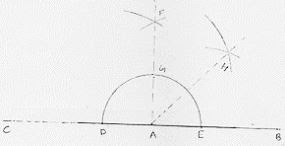Steps of construction :
1. Draw a line segment AB and produce BA to point C.
2. With center A and any radius drawn an arc which intersect AC at D and AB at E.
3. With center D and E and radius more than 1/2 DE, draw arcs cutting each other at F.
4. Join FA which intersect arc in (2) at G.
5. With centers G and E and radius more than  1/2 GE, draw arcs cutting each other at H.
6. Join HA
∴ ∠HAB = 45°

(ii)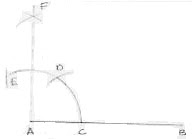Steps of construction :
1. Draw a line segment AB.
2. With center A and any radius draw in arc which intersect AB at C.
3. With center C and same radius draw an arc which intersects previous arc at D.
4. With centers D same radius draw an arc which intersects are in (2) at E.
5. With centers E and D same radius more than 1/2 ED draw an arc cutting each other at F.
6. Join FA
∠FAB = 90°

11. Construct the angles of the following measurements :
(i) 30°
(ii) 75°
(iii) 105°
(iv) 135°
(v) 15°
(vi) 45°/2
Solution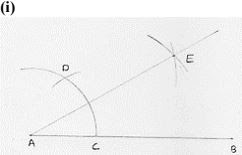Steps of construction :
1. Draw a line segment AB.
2. With center A and any radius, draw an arc which intersect AB at C.
3. With center C and same radius, draw an arc which intersects previous arc at D.
4. With centers D and C and radius more than 1/2 DC, draw arcs intersecting each other at E.
5. Join EA
∴ ∠EAB = 30°

(ii)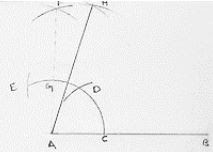Steps of construction :
1. Draw a line segment AB.
2. With center A any radius, draw an arc which intersect AB at C.
3. With center C and same radius, draw an arc which intersects previous arc at D.
4. With center D and same radius, draw an arc which intersects are in (2) at E.
5. With centers E and D and radius more than  1/2 ED, draw arcs intersecting each other at F.
6. Join FA which intersects arc in (2) at G
7. With centers G and D, and radius more than 1/2 GD, draw arcs intersecting each other at H.
8. Join HA
∴ ∠HAB = 75°

(iii)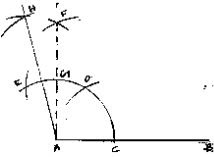Steps of construction :
1. 1Draw a line segment AB.
2. With center A and any radius, draw an arc intersect AB at C.
3. With center C and same radius, draw an arc which intersects previous arc at D.
4. With center D and same radius, draw an arc which interest are in (2) at E.
5. With centers E and D and radius more than 1/2 ED, draw arcs intersecting each other at F.
6. Join FA which intersects arc in (2) at E.
7. With centers E and G, and radius more than half of EG, draw arcs intersecting each other at H.
8. Join HA
∠HAB = 105°

(iv)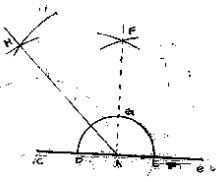Steps of construction :
1. Draw a line segment AB and produce BA to point C
2. With center A and any radius, draw an arc which intersect AC to D and AB at E.
3. With center D and E and radius more than half of DE, draw two arcs which intersects each other at F.
4. Join FA which intersect the arc in (2) at G.
5. With center G and D radius more than 1/2 GD, draw two arcs which intersect each other at H.
6. Join HA
∠HAB = 135°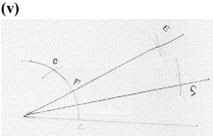Steps of construction :
1. Draw a line segment AB
2. With center A and any radius, draw an arc which intersects previous arc at C .
3. With center C and same radius, draw an arc which intersect previous arc at D .
4. With center D and C radius more than half of DC draw arcs intersecting each other at E.
5. Join EA which intersects arc in (2) at F.
6. With centers F and C and radius more than 1/2 FC, draw arcs intersecting each other.
7. Join GA
∴ ∠GAB = 15°

(vi)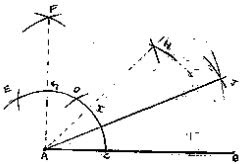Steps of construction :
1. Draw a line segment AB
2. With center A and any radius, draw an arc which intersects AB at C .
3. With center C and same radius, draw an arc which intersect previous arc at D.
4. With center D and same radius, draw an arc which intersects arc in (2) at E.
5. With center E and D and radius more than half of ED, draw arcs intersecting each
6. other at F.
7. Join FA which intersects arc in (2) at G.
8. With center G and C and radius more than half of GC, draw arcs intersecting each other at H.
9. Join HA which intersects arc in (2) at 1.
10. With centers I and C and radius more than half of IC, draw arcs intersecting each other.
11. Join JA
∴ ∠JAB = 45°/2